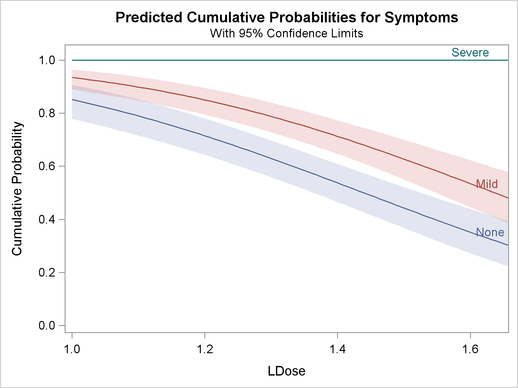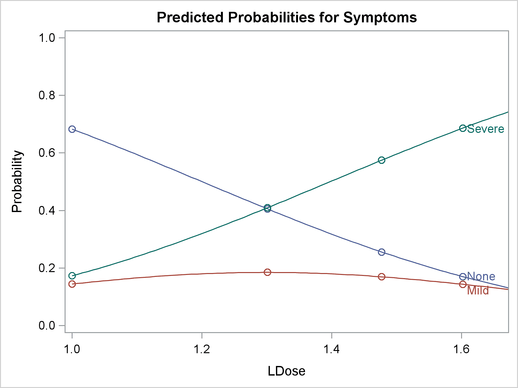## Example 74.2 Multilevel Response

In this example, two preparations, a standard preparation and a test preparation, are each given at several dose levels to groups of insects. The symptoms are recorded for each insect within each group, and two multilevel probit models are fit. Because the natural sort order of the three levels is not the same as the response order, the ORDER=DATA option is specified in the PROC PROBIT statement to get the desired order. The following statements fit two models:

```data multi;
input Prep \$ Dose Symptoms \$ N;
LDose=log10(Dose);
if Prep='test' then PrepDose=LDose;
else PrepDose=0;
datalines;
stand     10      None       33
stand     10      Mild        7
stand     10      Severe     10
stand     20      None       17
stand     20      Mild       13
stand     20      Severe     17
stand     30      None       14
stand     30      Mild        3
stand     30      Severe     28
stand     40      None        9
stand     40      Mild        8
stand     40      Severe     32
test      10      None       44
test      10      Mild        6
test      10      Severe      0
test      20      None       32
test      20      Mild       10
test      20      Severe     12
test      30      None       23
test      30      Mild        7
test      30      Severe     21
test      40      None       16
test      40      Mild        6
test      40      Severe     19
;
```
```proc probit order=data data=multi;
class Prep Symptoms;
nonpara: model Symptoms=Prep LDose PrepDose / lackfit;
weight N;
run;
```
```proc probit order=data data=multi ;
class Prep Symptoms;
parallel: model Symptoms=Prep LDose / lackfit;
weight N;
run;
```

Results of these two models are shown in Output 74.2.1 and Output 74.2.2. The first model allows for nonparallelism between the dose response curves for the two preparations by inclusion of an interaction between Prep and LDose. The interaction term is labeled PrepDose in the "Analysis of Parameter Estimates" table. The results of this first model indicate that the parameter for the interaction term is not significant, having a Wald chi-square of 0.73. Also, since the first model is a generalization of the second, a likelihood ratio test statistic for this same parameter can be obtained by multiplying the difference in log likelihoods between the two models by 2. The value obtained,, is 0.73. This is in close agreement with the Wald chi-square from the first model. The lack-of-fit test statistics for the two models do not indicate a problem with either fit.

Output 74.2.1 Multilevel Response: Nonparallel Analysis
The Probit Procedure

Model Information
Data Set WORK.MULTI
Dependent Variable Symptoms
Weight Variable N
Number of Observations 23
Name of Distribution Normal
Log Likelihood -345.9401767

Class Level Information
Name Levels Values
Prep 2 stand test
Symptoms 3 None Mild Severe

Analysis of Maximum Likelihood Parameter Estimates
Parameter   DF Estimate Standard Error 95% Confidence Limits Chi-Square Pr > ChiSq
Intercept   1 3.8080 0.6252 2.5827 5.0333 37.10 <.0001
Intercept2   1 0.4684 0.0559 0.3589 0.5780 70.19 <.0001
Prep stand 1 -1.2573 0.8190 -2.8624 0.3479 2.36 0.1247
Prep test 0 0.0000 . . . . .
LDose   1 -2.1512 0.3909 -2.9173 -1.3851 30.29 <.0001
PrepDose   1 -0.5072 0.5945 -1.6724 0.6580 0.73 0.3935

Output 74.2.2 Multilevel Response: Parallel Analysis
The Probit Procedure

Model Information
Data Set WORK.MULTI
Dependent Variable Symptoms
Weight Variable N
Number of Observations 23
Name of Distribution Normal
Log Likelihood -346.306141

Class Level Information
Name Levels Values
Prep 2 stand test
Symptoms 3 None Mild Severe

Analysis of Maximum Likelihood Parameter Estimates
Parameter   DF Estimate Standard Error 95% Confidence Limits Chi-Square Pr > ChiSq
Intercept   1 3.4148 0.4126 2.6061 4.2235 68.50 <.0001
Intercept2   1 0.4678 0.0558 0.3584 0.5772 70.19 <.0001
Prep stand 1 -0.5675 0.1259 -0.8142 -0.3208 20.33 <.0001
Prep test 0 0.0000 . . . . .
LDose   1 -2.3721 0.2949 -2.9502 -1.7940 64.68 <.0001

The negative coefficient associated with LDose indicates that the probability of having no symptoms (Symptoms=’None’) or no or mild symptoms (Symptoms=’None’ or Symptoms=’Mild’) decreases as LDose increases; that is, the probability of a severe symptom increases with LDose. This association is apparent for both treatment groups.

The negative coefficient associated with the standard treatment group (Prep = stand) indicates that the standard treatment is associated with more severe symptoms across all Ldose values.

The following statements use the PLOTS= option to create the plot shown in Output 74.2.3 and Output 74.2.4. Output 74.2.3 is the plot of the probabilities of the response taking on individual levels as a function of LDose. Since there are two covariates, LDose and Prep, the value of the classification variable Prep is fixed at the highest level, test. Instead of individual response level probabilities, the CDFPLOT option creates the plot of the cumulative response probabilities with confidence limits shown in Output 74.2.4.

```ods graphics on;

proc probit data=multi order=data
plots=(predpplot(level=("None" "Mild" "Severe"))
cdfplot(level=("None" "Mild" "Severe")));
class Prep Symptoms;
parallel: model Symptoms=Prep LDose / lackfit;
weight N;
run;

ods graphics off;
```

Output 74.2.3 Plot of Predicted Probabilities for the Test Preparation GroupOutput 74.2.4 Plot of Predicted Cumulative Probabilities for the Test Preparation GroupThe following statements use the XDATA= data set to create plots of predicted probabilities and cumulative probabilities with Prep set to the stand level. The resulting plots are shown in Output 74.2.5 and Output 74.2.6.

```data xrow;
input Prep \$ Dose Symptoms \$ N;
LDose=log10(Dose);
datalines;
stand     40      Severe     32
run;

ods graphics on;

proc probit data=multi order=data xdata=xrow
plots=(predpplot(level=("None" "Mild" "Severe"))
cdfplot(level=("None" "Mild" "Severe")));
class Prep Symptoms;
parallel: model Symptoms=Prep LDose / lackfit;
weight N;
run;

ods graphics off;
```

Output 74.2.5 Plot of Predicted Probabilities for the Standard Preparation GroupOutput 74.2.6 Plot of Predicted Cumulative Probabilities for the Standard Preparation Group